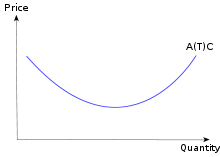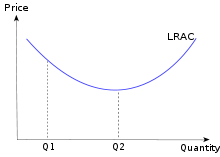• Have any questions?
• +91-9540-660064
• hahassignment@gmail.com

# Cost Curves Assignment help

In economics, a cost curve is a graph of the costs of production as a function of total quantity produced. In a free market economy, productively efficient firms use these curves to find the optimal point of production (minimising cost), and profit maximizing firms can use them to decide output quantities to achieve those aims. There are various types of cost curves, all related to each other, including total and average cost curves, and marginal ("for each additional unit") cost curves, which are the equal to the differential of the total cost curves. Some are applicable to the short run, others to the long run.

• Short-run average variable cost curve (SRAVC).
• Short-run average total cost curve (SRATC or SRAC).
• Long-run average cost curve (LRAC).
• Short-run marginal cost curve (SRMC).
• Long-run marginal cost curve (LRMC).
• Graphing cost curves together.
• Cost curves and production functions.

Short-run average variable cost curve (SRAVC)
Average variable cost (which is a short-run concept) is the variable cost (typically labor cost) per unit of output: SRAVC = wL / Q where w is the wage rate, L is the quantity of labor used, and Q is the quantity of output produced. The SRAVC curve plots the short-run average variable cost against the level of output, and is typically U-shaped.

### Short-run average total cost curve (SRATC or SRAC)

The average total cost curve is constructed to capture the relation between cost per unit of output and the level of output, ceteris paribus. A perfectly competitive and productively efficient firm organizes its factors of production in such a way that the average cost of production is at the lowest point. In the short run, when at least one factor of production is fixed, this occurs at the output level where it has enjoyed all possible average cost gains from increasing production. This is at the minimum point in the diagram on the right.

Short-run total cost is given by

STC = PKK+PLL,

where PK is the unit price of using physical capital per unit time, PL is the unit price of labor per unit time (the wage rate), K is the quantity of physical capital used, and L is the quantity of labor used. From this we obtain short-run average cost, denoted either SATC or SAC, as STC / Q:

SRATC or SRAC = PKK/Q + PLL/Q = PK / APK + PL / APL,

where APK = Q/K is the average product of capital and APL = Q/L is the average product of labor.

Short run average cost equals average fixed costs plus average variable costs. Average fixed cost continuously falls as production increases in the short run, because K is fixed in the short run. The shape of the average variable cost curve is directly determined by increasing and then diminishing marginal returns to the variable input (conventionally labor)### Long-run average cost curve (LRAC)

The long-run average cost curve depicts the cost per unit of output in the long run—that is, when all productive inputs' usage levels can be varied. All points on the line represent least-cost factor combinations; points above the line are attainable but unwise, while points below are unattainable given present factors of production. The behavioral assumption underlying the curve is that the producer will select the combination of inputs that will produce a given output at the lowest possible cost.In a long-run perfectly competitive environment, the equilibrium level of output corresponds to the minimum efficient scale, marked as Q2 in the diagram. This is due to the zero-profit requirement of a perfectly competitive equilibrium. This result, which implies production is at a level corresponding to the lowest possible average cost, does not imply that production levels other than that at the minimum point are not efficient. All points along the LRAC are productively efficient, by definition, but not all are equilibrium points in a long-run perfectly competitive environment. In some industries, the bottom of the LRAC curve is large in comparison to market size (that is to say, for all intents and purposes, it is always declining and economies of scale exist indefinitely). This means that the largest firm tends to have a cost advantage, and the industry tends naturally to become a monopoly, and hence is called a natural monopoly. Natural monopolies tend to exist in industries with high capital costs in relation to variable costs, such as water supply and electricity supply

### Short-run marginal cost curve (SRMC)

A short-run marginal cost curve graphically represents the relation between marginal (i.e., incremental) cost incurred by a firm in the short-run production of a good or service and the quantity of output produced. This curve is constructed to capture the relation between marginal cost and the level of output, holding other variables, like technology and resource prices, constant. The marginal cost curve is U-shaped. Marginal cost is relatively high at small quantities of output; then as production increases, marginal cost declines, reaches a minimum value, then rises. The marginal cost is shown in relation to marginal revenue, the incremental amount of sales revenue that an additional unit of the product or service will bring to the firm. This shape of the marginal cost curve is directly attributable to increasing, then decreasing marginal returns (and the law of diminishing marginal returns). Marginal cost equals w/MPL. For most production processes the marginal product of labor initially rises, reaches a maximum value and then continuously falls as production increases. Thus marginal cost initially falls, reaches a minimum value and then increases. The marginal cost curve intersects both the average variable cost curve and (short-run) average total cost curve at their minimum points. When the marginal cost curve is above an average cost curve the average curve is rising. When the marginal costs curve is below an average curve the average curve is falling. This relation holds regardless of whether the marginal curve is rising or falling.### Long-run marginal cost curve (LRMC)

The long-run marginal cost curve shows for each unit of output the added total cost incurred in the long run, that is, the conceptual period when all factors of production are variable so as minimize long-run average total cost. Stated otherwise, LRMC is the minimum increase in total cost associated with an increase of one unit of output when all inputs are variable. The long-run marginal cost curve is shaped by economies and diseconomies of scale, a long-run concept, rather than the law of diminishing marginal returns, which is a short-run concept. The long-run marginal cost curve tends to be flatter than its short-run counterpart due to increased input flexibility as to cost minimization. The long-run marginal cost curve intersects the long-run average cost curve at the minimum point of the latter.:208 When long-run marginal costs are below long-run average costs, long-run average costs are falling (as to additional units of output). When long-run marginal costs are above long run average costs, average costs are rising. Long-run marginal cost equals short run marginal-cost at the least-long-run-average-cost level of production. LRMC is the slope of the LR total-cost function.

In case you face any problem or have any query please email us at :-info@homeworkassignmenthelp.com

### Submit us an Assignment:

Our tutors start working only after the payment is made, to ensure that we are doing work only for serious clients and also our solution meets the required standard.

### Getting homework help was never so easy you just need to follow following steps:

• Send us you Other Assignment or problem through email
• Specify the required format such as Word, Excel, Notepad, PDF
• Give us a deadline when you need the assignment completed along with the Time Zone.
(for example: EST, Australian GMT etc)
• Send documents related to your assignment which can help our tutors to provide a better work,
any example or format you want the solutions to be in.
• Our tutors will review the assignment sent by you and if all the required information is there we will
send you the price quoted by our tutor along with the time needed to solve the assignment
• You can pay us through paypal or credit card.
• After receiving the payment tutors start working on your assignment.
• Finally, we deliver the solutions and get a feedback from you regarding our work

In case you face any problem or have any query please email us at :- info@homeworkassignmenthelp.com